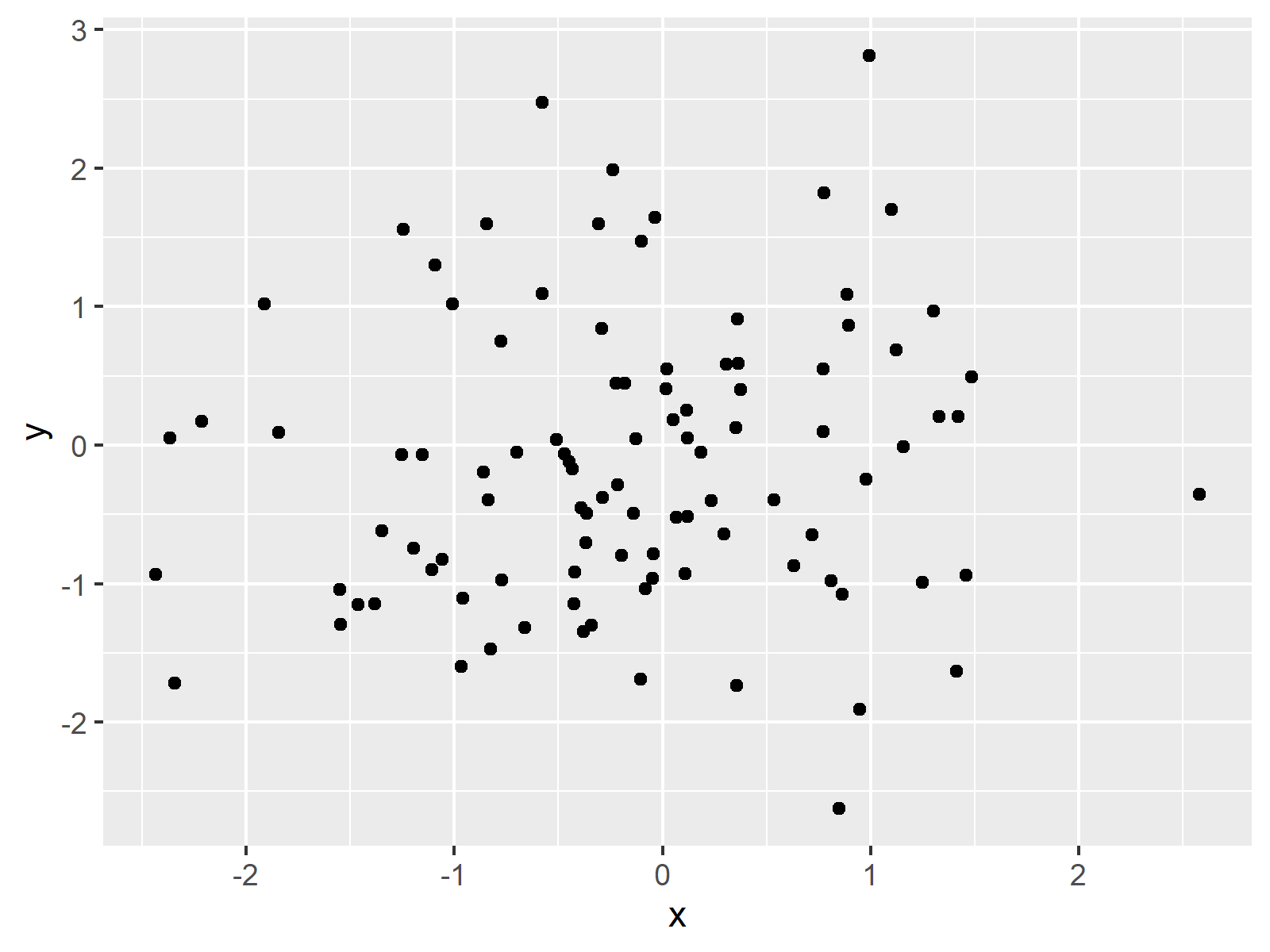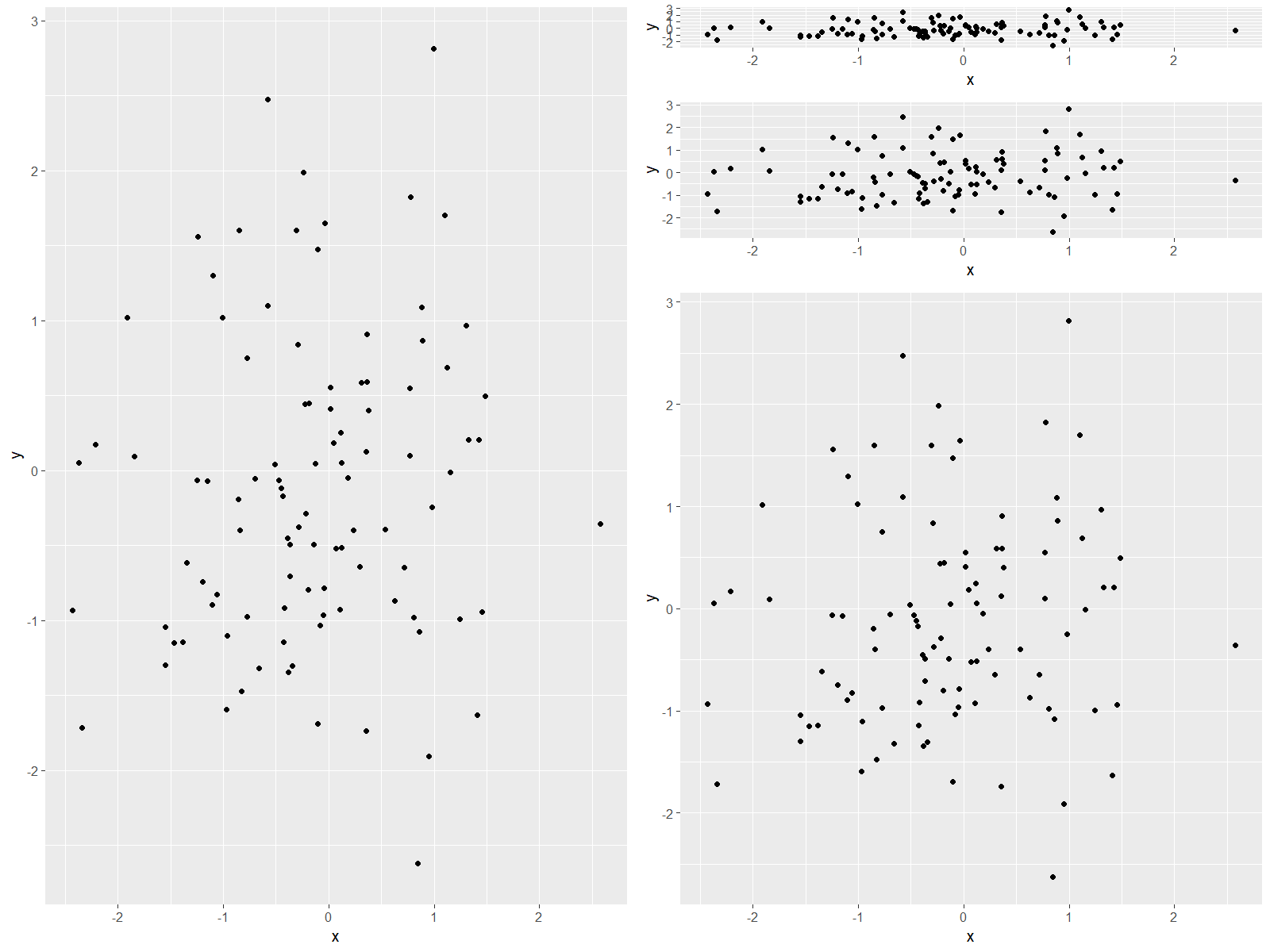# Draw Unbalanced Grid of ggplot2 Plots in R (Example)

This page explains how to create a grid of plots with different sizes in the R programming language.

The tutorial contains this content:

Let’s just jump right in…

## Example Data

We’ll use the following data frame as example data:

```set.seed(963) # Create example data data <- data.frame(x = rnorm(100), y = rnorm(100)) head(data) # Print first rows of data # x y # 1 -0.3814289 -1.34755690 # 2 1.0976566 1.70212897 # 3 0.1141007 0.25060268 # 4 1.4211450 0.20545551 # 5 0.1847765 -0.05066872 # 6 1.4850370 0.49485481```

Our data consists of 100 rows and two numeric columns x and y.

Furthermore, we need to install and load the ggplot2 package

```install.packages("ggplot2") # Install and load ggplot2 library("ggplot2")```

…as well as the gridExtra package:

```install.packages("gridExtra") # Install and load gridExtra library("gridExtra")```

Now, we can draw a plot with the functions of the ggplot2 package as shown below:

```ggp <- ggplot(data, aes(x, y)) + # Create ggplot2 scatterplot geom_point() ggp # Draw plot```Figure 1: Simple ggplot2 Scatterplot.

Figure 1 visualizes how our example plot looks like: It’s a basic scatterplot.

In the following, I’ll explain how to draw this scatterplot multiple times side-by-side – each time with a different size. So keep on reading…

## Example: Draw Multiple Plots with Different Size

If we want to create a grid of plots with different sizes, we can use the grid.arrange and arrangeGrob functions of the gridExtra package. Within these functions, we can specify the number of columns and rows of our grid as well as the height and width of each graph:

```grid.arrange(ggp, # Arrange plot in grid arrangeGrob(ggp, ggp, ggp, heights = c(0.1, 0.2, 0.7)), ncol = 2)```Figure 2: Multiple ggplot2 Plots in an Unbalanced Grid.

Figure 2 shows the output of the previous R code. As you can see, we created a grid consisting of five plots with different sizes.

## Video & Further Resources

Would you like to learn more about the plotting of data in grids? Then you might want to have a look at the following video of my YouTube channel. In the video, I illustrate the R code of this tutorial.

Please accept YouTube cookies to play this video. By accepting you will be accessing content from YouTube, a service provided by an external third party.If you accept this notice, your choice will be saved and the page will refresh.

In addition, I can recommend to have a look at the related articles of this website. I have released numerous articles already:

In this tutorial you learned how to obtain an unbalanced layout of graphics in the R programming language. Please let me know in the comments section, in case you have any further questions.

Subscribe to the Statistics Globe Newsletter